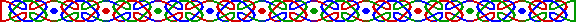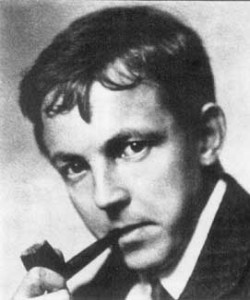英國數學家哈代 (Godfrey Harold Hardy 1877-1947)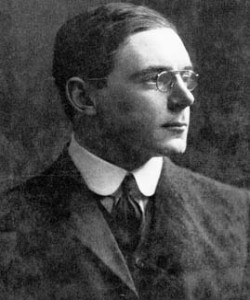英國數學家李特伍德 (John Edenser Littlewood 1885-1977)

(照片取自「The MacTutor History of Mathematics Achieve」http://www-gap.dcs.st-and.ac.uk/~history/ )(3,5)、(5,7)、(11,13)、(17,19)、(29,31)等，這些組合中的兩個素數均相 2 ，我們稱為一對對的孿生素數 (Twin Primes)。孿生即雙胞胎，這些素數組一對一對相鄰，尢如雙生嬰兒般。用數學的用詞即若 p 為素數， p+2 也是素數。

 孿生素數 數位 發現者 發現年份 2003663613 * 2195000 ± 1 58711 維達 (Eric Vautier) / 麥杰本 (Patrick W. McKibbon) / 杰華賓高 (Dmitri Gribenko) 2007 194772106074315 * 2171960 ± 1 51780 索坦．查韋 (Zoltan Jarai) / 法加斯 (Gabor Farkas) / 莎織波克(Timea Csajbok) / 加斯沙 (Janos Kasza) / 安杜．查韋 (Antal Jarai) 2007 100314512544015 * 2171960 ± 1 51780 索坦．查韋 (Zoltan Jarai) / 法加斯 (Gabor Farkas) / 莎織波克(Timea Csajbok) / 加斯沙 (Janos Kasza) / 安杜．查韋 (Antal Jarai) 2006 16869987339975 * 2171960 ± 1 51779 索坦．查韋 (Zoltan Jarai) / 法加斯 (Gabor Farkas) / 莎織波克(Timea Csajbok) / 加斯沙 (Janos Kasza) / 安杜．查韋 (Antal Jarai) 2005 33218925 * 2169690 ± 1 51090 柏比 (Daniel Papp) / 加洛特 (Yves Gallot) 2002 60194061 * 2114689 ± 1 34533 安達碧基 (David Underbakke) 2002 108615 * 2110342 ± 1 33222 查達菲特 (Chris Chatfield) 2008 1765199373 * 2107520 ± 1 32376 麥伊赫頓 (James McElhatton) / 加洛特 (Yves Gallot) 2002 318032361 * 2107001 ± 1 32220 安達碧基 (David Underbakke) / 卡莫迪(Phil Carmody) 2001 1046619117 * 2100000 ± 1 30113 巴恩斯 (Gary Barnes) 20071949年，基文特 (P. A. Clement) 對孿生素數作了以下的描述：

(n-1)! + 1 = 0 (mod n)

4(n-1)! + 2 = 0 (mod n+2)

4(n+1)! + 2*n*(n+1) = 0 (mod n+2)

4[(n+1)! + 1] + 2n2 + 2n - 4 = 0 (mod n+2)

4[(n+1)! + 1] + (n+2) (2n-2) = 0 (mod n+2)

(n-1)! + 1 = 0 (mod n) 及 (n+1)! + 1 = 0 (mod n+2)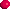除了 (3,5) 一組外，所有孿生素數的和均是 12 的倍數 (Multiple)。

5 + 7 = 12、11 + 13 = 24 = 2 * 12、17 + 19 = 36 = 3 * 12、29 + 31 = 60 = 5 * 12、......Caldwell, C. "The Top Twenty: Twin Primes." http://primes.utm.edu/top20/page.php?id=1.

Guy, R. K. "Gaps between Primes. Twin Primes." §A8 in Unsolved Problems in Number Theory, 2nd ed. New York: Springer-Verlag, pp. 19-23, 1994.

Ribenboim, P. "The Little Book of Bigger Prime" , New York: Springer-Verlag, 1991

Wells, D. The Penguin Dictionary of Curious and Interesting Numbers. Middlesex, England: Penguin Books, p. 41, 1986.

Weisstein, E. W. "Chen Prime." From MathWorld. http://mathworld.wolfram.com/ChenPrime.html.

Weisstein, E. W. "Twin Primes." From MathWorld. http://mathworld.wolfram.com/TwinPrimes.html.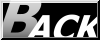Hosted by www.Geocities.ws